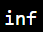# What is Float in Python?

Float is one of the basic built-in data types in Python, paired with int and diverse data types. The float function in Python’s standard library transforms an integer or a string of numbers into a float data type. When a string is regarded as valid for converting to a float, the following rules apply.

• The string must only include integers.

• Mathematical operators can also be applied between the numbers.

• The string might be NaN or inf

• White spaces at the start and finish are always ignored.

Examples for working of “float()”:

As an integer:

``````print(float(77.9))
``````

Output: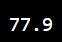Floating point:

``````print(float(8))
``````

Output: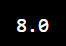Floating type string:

``````print(float("-16.54"))
``````

Output: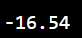For NaN:

``````print(float("NaN"))
``````

Output: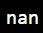For inf:

``````print(float("inf"))
``````

Output: